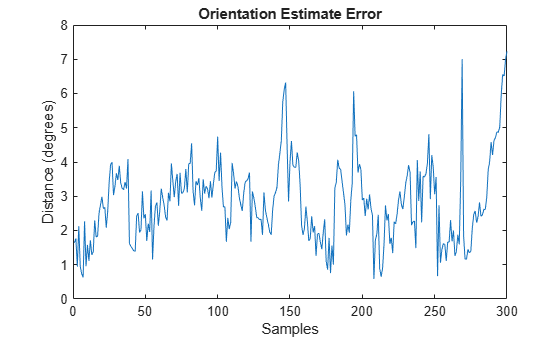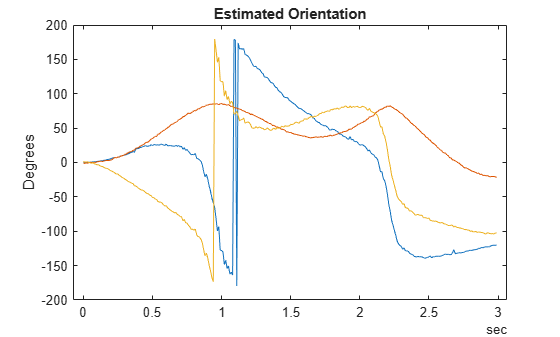# insEKF

Inertial Navigation Using Extended Kalman Filter

## Description

The `insEKF` object creates a continuous-discrete extended Kalman Filter (EKF), in which the state prediction uses a continuous-time model and the state correction uses a discrete-time model. The filter uses data from inertial sensors to estimate platform states such as position, velocity, and orientation. The toolbox provides a few sensor models, such as `insAccelerometer`, `insGyroscope`, `insGPS`, and `insMagnetometer`, that you can use to enable the corresponding measurements in the EKF. You can also customize your own sensor models by inheriting from the `positioning.insSensorModel` interface class. The toolbox also provides motion models, such as `insMotionOrientation` and `insMotionPose`, that you can use to enable the corresponding state propagation in the EKF. You can also customize your own motion models by inheriting from the `positioning.insMotionModel` interface class.

## Creation

### Syntax

``filter = insEKF``
``filter = insEKF(sensor1,sensor2,...,sensorN)``
``filter = insEKF(___,motionModel)``
``filter = insEKF(___,options)``

### Description

example

````filter = insEKF` creates an `insEKF` filter object with default property values. With the default settings, the filter can estimate orientation by fusing accelerometer and gyroscope data. ```

example

````filter = insEKF(sensor1,sensor2,...,sensorN)` configures the filter to accept and fuse data from one or more sensors. The filter saves these sensors in its `Sensors` property. ```
````filter = insEKF(___,motionModel)` configures the filter to use the motion model to predict and estimate state, in addition to any combination of input arguments from previous syntaxes. The filter saves the specified motion model in the `MotionModel` property.```
````filter = insEKF(___,options)` configures the filter using the `insOptions` object `options`.```

## Properties

expand all

State vector of the extended Kalman filter, specified as an N-element real-valued vector. N is the dimension of the filter state, determined by the specific sensors and motion model used to construct the filter.

Note

In the `State` property, if a state variable named `Orientation` has a length of four, the object assumes it is a `quaternion`. In that case, the filter renormalizes the quaternion and ensures that the real part of the quaternion is always positive.

Data Types: `single` | `double`

State error covariance for the extended Kalman filter, specified as an N-by-N real-valued positive-definite matrix. N is the dimension of the state, specified in the `State` property of the filter.

Data Types: `single` | `double`

Additive process noise for the extended Kalman filter, specified as an N-by-N real-valued positive definite matrix. N is the dimension of the state, specified in the `State` of the filter.

Data Types: `single` | `double`

Motion model used in the extended Kalman filter, specified as an `insMotionOrientation` object , an `insMotionPose` object, or an object inheriting from the `positioning.INSMotionModel` interface class. Specify a motion model using the `motionModel` input argument.

Data Types: `object`

Sensors fused in the extended Kalman filter, specified as a cell array of inertial sensor objects. An inertial sensor object is one of these objects:

Data Types: `cell`

Names of the sensors, specified as a cell array of character vectors. By default, the filter names the sensors using the format `'sensorname_n'`, where `sensorname` is the name of the sensor, such as `Accelerometer`, and `n` is the index for additional sensors of the same type.

To customize the sensor names, specify the `options` input when constructing the filter.

Example: ```{'Accelerometer' 'Accelerometer_1' 'Accelerometer_2' 'Gyroscope'}```

Data Types: `cell`

Reference frame of the extended Kalman filter, specified as `"NED"` for the north-east-down frame or `"ENU"` for the east-north-up frame.

To specify the reference frame as `"ENU"`, specify the `options` input when constructing the filter.

Data Types: `char` | `string`

## Object Functions

 `predict` Predict state estimates forward in time for `insEKF` `fuse` Fuse sensor data for state estimation in `insEKF` `residual` Residual and residual covariance from state measurement for `insEKF` `correct` Correct state estimates in `insEKF` using direct state measurements `stateparts` Get and set part of state vector in `insEKF` `statecovparts` Get and set part of state covariance matrix in `insEKF` `stateinfo` State vector information for `insEKF` `estimateStates` Batch fusion of sensor data `tune` Tune `insEKF` parameters to reduce estimation error `createTunerCostTemplate` Create template of tuner cost function `tunerCostFcnParam` First parameter example for tuning cost function `copy` Create copy of `insEKF` `reset` Reset states for `insEKF`

## Examples

collapse all

Create a default `insEKF` object. By default, the filter fuses the measurement data from an accelerometer and a gyroscope assuming orientation-only motion.

`filter1 = insEKF`
```filter1 = insEKF with properties: State: [13x1 double] StateCovariance: [13x13 double] AdditiveProcessNoise: [13x13 double] MotionModel: [1x1 insMotionOrientation] Sensors: {[1x1 insAccelerometer] [1x1 insGyroscope]} SensorNames: {'Accelerometer' 'Gyroscope'} ReferenceFrame: 'NED' ```

Create a second `insEKF` object that fuses data from an accelerometer, a gyroscope, and a magnetometer, as well as models both rotational motion and translational motion.

`filter2 = insEKF(insAccelerometer,insGyroscope,insMagnetometer,insMotionPose)`
```filter2 = insEKF with properties: State: [28x1 double] StateCovariance: [28x28 double] AdditiveProcessNoise: [28x28 double] MotionModel: [1x1 insMotionPose] Sensors: {1x3 cell} SensorNames: {'Accelerometer' 'Gyroscope' 'Magnetometer'} ReferenceFrame: 'NED' ```

Create a third `insEKF` object that fuses data from a gyroscope and a GPS. Specify the reference frame of the filter as the east-north-up (ENU) frame. Note that the motion model that the filter uses is the `insMotionPose` object because a GPS measures platform positions.

```option = insOptions(ReferenceFrame="ENU"); filter3 = insEKF(insGyroscope,insGPS,option)```
```filter3 = insEKF with properties: State: [19x1 double] StateCovariance: [19x19 double] AdditiveProcessNoise: [19x19 double] MotionModel: [1x1 insMotionPose] Sensors: {[1x1 insGyroscope] [1x1 insGPS]} SensorNames: {'Gyroscope' 'GPS'} ReferenceFrame: 'ENU' ```

Load measurement data from an accelerometer and a gyroscope.

`load("accelGyroINSEKFData.mat");`

Create an `insEKF` filter object. Specify the orientation part of the state in the filter using the initial orientation from the measurement data. Specify the diagonal elements of the state estimate error covariance matrix corresponding to the orientation state as `0.01`.

```accel = insAccelerometer; gyro = insGyroscope; filt = insEKF(accel,gyro); stateparts(filt,"Orientation",compact(ld.initOrient)); statecovparts(filt,"Orientation",1e-2);```

Specify the measurement noise and the additive process noise. You can obtain these values by using the `tune` object function of the filter object.

```accNoise = 0.1739; gyroNoise = 1.1129; processNoise = diag([ ... 2.8586 1.3718 0.8956 3.2148 4.3574 2.5411 3.2148 0.5465 0.2811 ... 1.7149 0.1739 0.7752 0.1739]); filt.AdditiveProcessNoise = processNoise;```

Sequentially fuse the measurement data using the `predict` and `fuse` object functions of the filter object.

```N = size(ld.sensorData,1); estOrient = quaternion.zeros(N,1); dt = seconds(diff(ld.sensorData.Properties.RowTimes)); for ii = 1:N if ii ~= 1 % Step forward in time. predict(filt,dt(ii-1)); end % Fuse accelerometer data. fuse(filt,accel,ld.sensorData.Accelerometer(ii,:),accNoise); % Fuse gyroscope data. fuse(filt,gyro,ld.sensorData.Gyroscope(ii,:),gyroNoise); % Extract the orientation state estimate using the stateparts object % function. estOrient(ii) = quaternion(stateparts(filt,"Orientation")); end```

Visualize the estimate error, in quaternion distance, using the `dist` object function of the `quaternion` object.

```figure plot(rad2deg(dist(estOrient,ld.groundTruth.Orientation))) xlabel("Samples") ylabel("Distance (degrees)") title("Orientation Estimate Error")```Load measurement data from an accelerometer and a gyroscope.

`load("accelGyroINSEKFData.mat");`

Create an `insEKF` filter object. Specify the orientation part of the state in the filter using the initial orientation from the measurement data. Specify the diagonal elements of the state estimate error covariance matrix corresponding to the orientation state as `0.01`.

```filt = insEKF; stateparts(filt,"Orientation",compact(ld.initOrient)); statecovparts(filt,"Orientation",1e-2);```

Specify the measurement noise and the additive process noise. You can obtain these values by using the `tune` object function of the filter object.

```measureNoise = struct("AccelerometerNoise", 0.1739, ... "GyroscopeNoise", 1.1129); processNoise = diag([ ... 2.8586 1.3718 0.8956 3.2148 4.3574 2.5411 3.2148 0.5465 0.2811 ... 1.7149 0.1739 0.7752 0.1739]); filt.AdditiveProcessNoise = processNoise;```

Batch-estimate the states using the `estimateStates` object function.

`estimates = estimateStates(filt,ld.sensorData,measureNoise);`

Visualize the estimate error, in quaternion distance, using the `dist` object function of the `quaternion` object.

```figure plot(rad2deg(dist(estimates.Orientation,ld.groundTruth.Orientation))) xlabel("Samples") ylabel("Distance (degrees)") title("Orientation Estimate Error")```## Version History

Introduced in R2022a